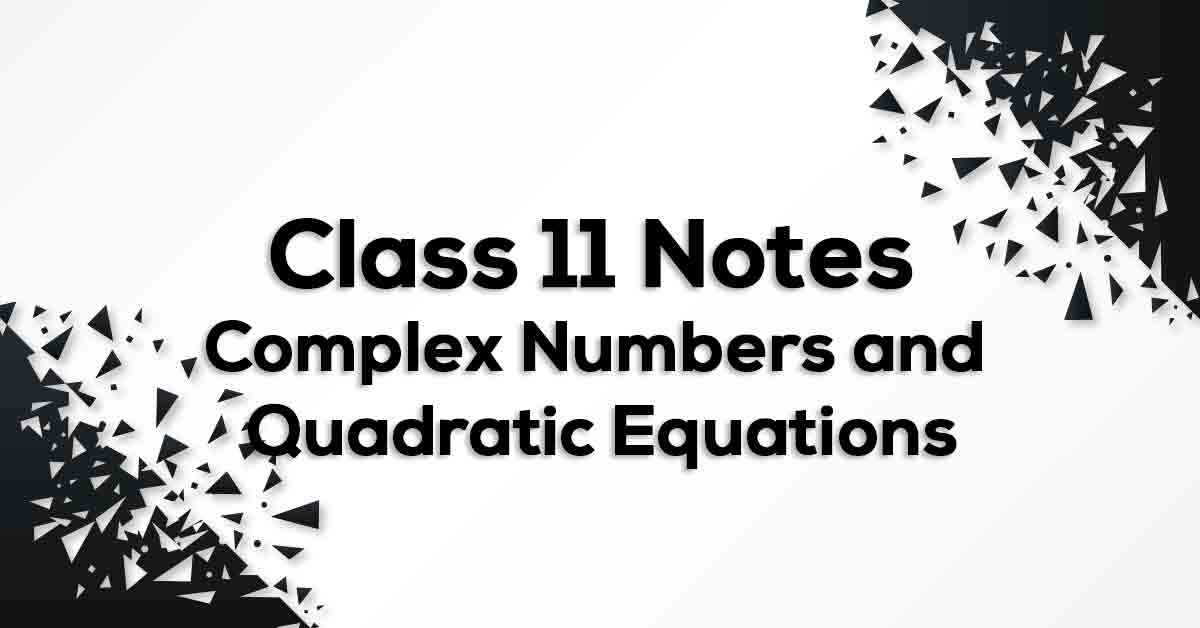Complex Numbers and Quadratic Equations Class 11 NotesClass 11 Math Chapter 5 Complex Numbers and Quadratic Equations Notes- PDF Download

Complex Numbers and Quadratic Equations Class 11 Notes is prepared strictly according to the NCERT Syllabus which not only reduces the pressure on the students but also, offer them a simple way to study or revise the chapter. These formulae are cumulated from past 15 years of examination material preferred by CBSE so that no important formulae should be left behind for the students to know and practice. Complex Numbers and Quadratic Equations Class 11 Notes would fuel your exam preparation and increase your self-confidence which would help you score maximum marks in the exam.

Define Complex Numbers and Quadratic Equations.

Complex number is of the form a +ib where a is real part and b is imaginary part. Here i = √ -1. The complex number x + iy  can be represented  geometrically as the unique point  P(x, y) in the XY-plane and vice-versa. Plane with complex number assigned to each of its point is called complex or Argand plane. The addition of complex numbers satisfies the following properties: Closure law: z1 + z2 = complex Number, Commutative law: z1 + z2 = z2 + z1, Associative law: (z1 + z2) + z3 = z1 + (z2 + z3). Additive identity: z + 0 = z. Additive inverse: z + (–z) = 0.